#The Wolfram Language:Fast Introduction for Programmers

Show additional notes for:
All sectionsGet Started

# Applying FunctionsView as Video

It's very common to want to "map" a function over multiple expressions:

In:=1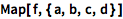`Map[f, {a, b, c, d}]`
Out=1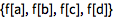Notes for Python programmers:

Map in the Wolfram Language is like map in Python, except that it can operate on arbitrary expression trees of any depth.

/@ ("slash at") is a short notation for Map:

In:=2`f /@ {a, b, c, d}`
Out=2Notes for Java programmers:

Map in the Wolfram Language works similarly to the Stream.map method in Java, except that Map can be applied to any kind of expression.

This uses a pure function:

In:=3`{#, #} & /@ {a, b, c, d}`
Out=3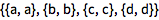Apply applies a function to multiple arguments:

In:=1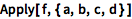`Apply[f, {a, b, c, d}]`
Out=1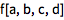Notes for Python programmers:

Apply in the Wolfram Language is similar to the unpacking operator * in Python.

Expressions have "levels"—corresponding to the number of indices needed to extract a part. Functions like Map can operate at specific levels.

Notes for Java programmers:

"Levels" are another name for dimensions of an array, but generalized for all symbolic expressions. Multi-dimensional operations like this are not built into Java and would normally require loops.

Notes for Python programmers:

"Levels" are like dimensions of an array, but generalized for all symbolic expressions. Python's array functions are typically set up only for one-dimensional arrays.

Map defaults to operate at level 1:

In:=1`Map[f, {{a, b}, {c, d}}]`
Out=1This operates only at level 2:

In:=2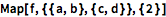`Map[f, {{a, b}, {c, d}}, {2}]`
Out=2@@ is equivalent to Apply, operating by default at level 0:

In:=1`f @@ {{a, b}, {c, d}}`
Out=1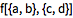@@@ means "apply at level 1":

In:=2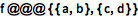`f @@@ {{a, b}, {c, d}}`
Out=2@ means ordinary function application:

In:=3`f@x`
Out=3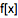Which expression evaluates to g[{a, b, c}]?

Which one of these expressions evaluates to {{f[a], f[b]}, {f[c], f[d]}}?

Which of the following evaluates to {{{f[a], f[b]}}, {{f[c], f[d]}}}?